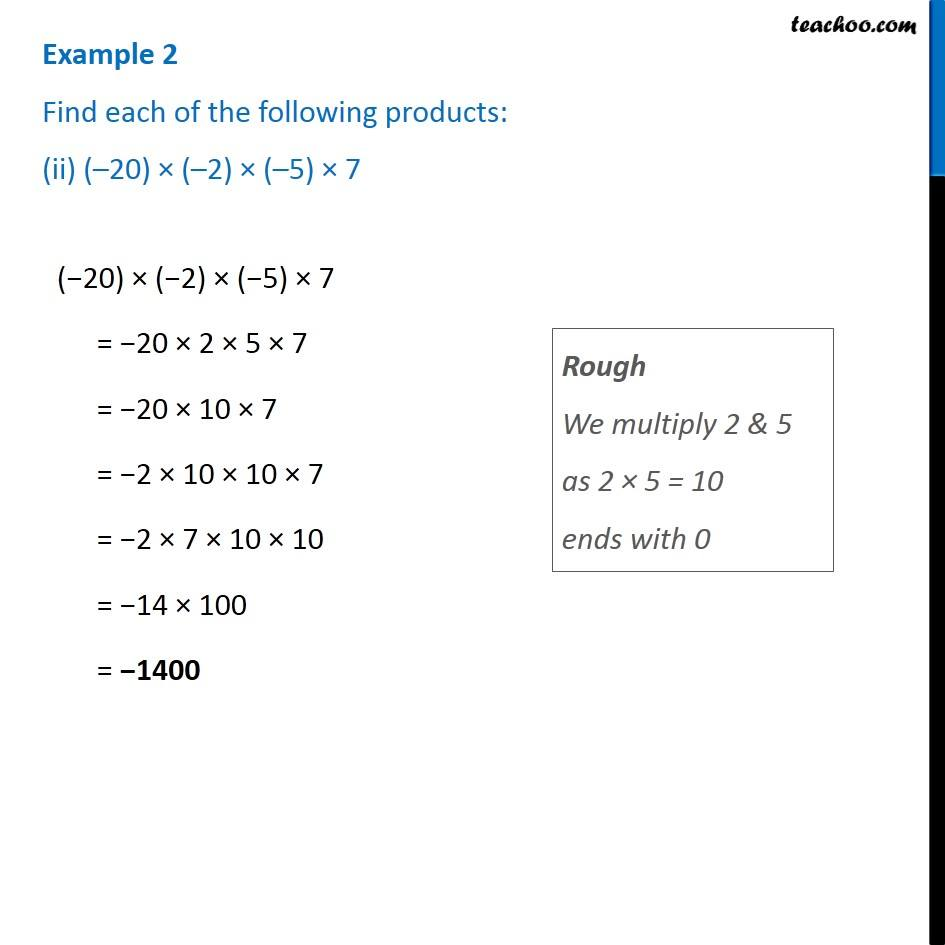Examples

Chapter 1 Class 7 Integers
Serial order wiseLearn in your speed, with individual attention - Teachoo Maths 1-on-1 Class

### Transcript

Example 2 Find each of the following products: (ii) ( 20) ( 2) ( 5) 7 ( 20) ( 2) ( 5) 7 = 20 2 5 7 = 20 10 7 = 2 10 10 7 = 2 7 10 10 = 14 100 = 1400 Rough We multiply 2 & 5 as 2 5 = 10 ends with 0